# Transmitting Data via Bluetooth Module and Arduino

How to transmit 0 to 9 digits using a Bluetooth module, a 7-segment display, and an Arduino.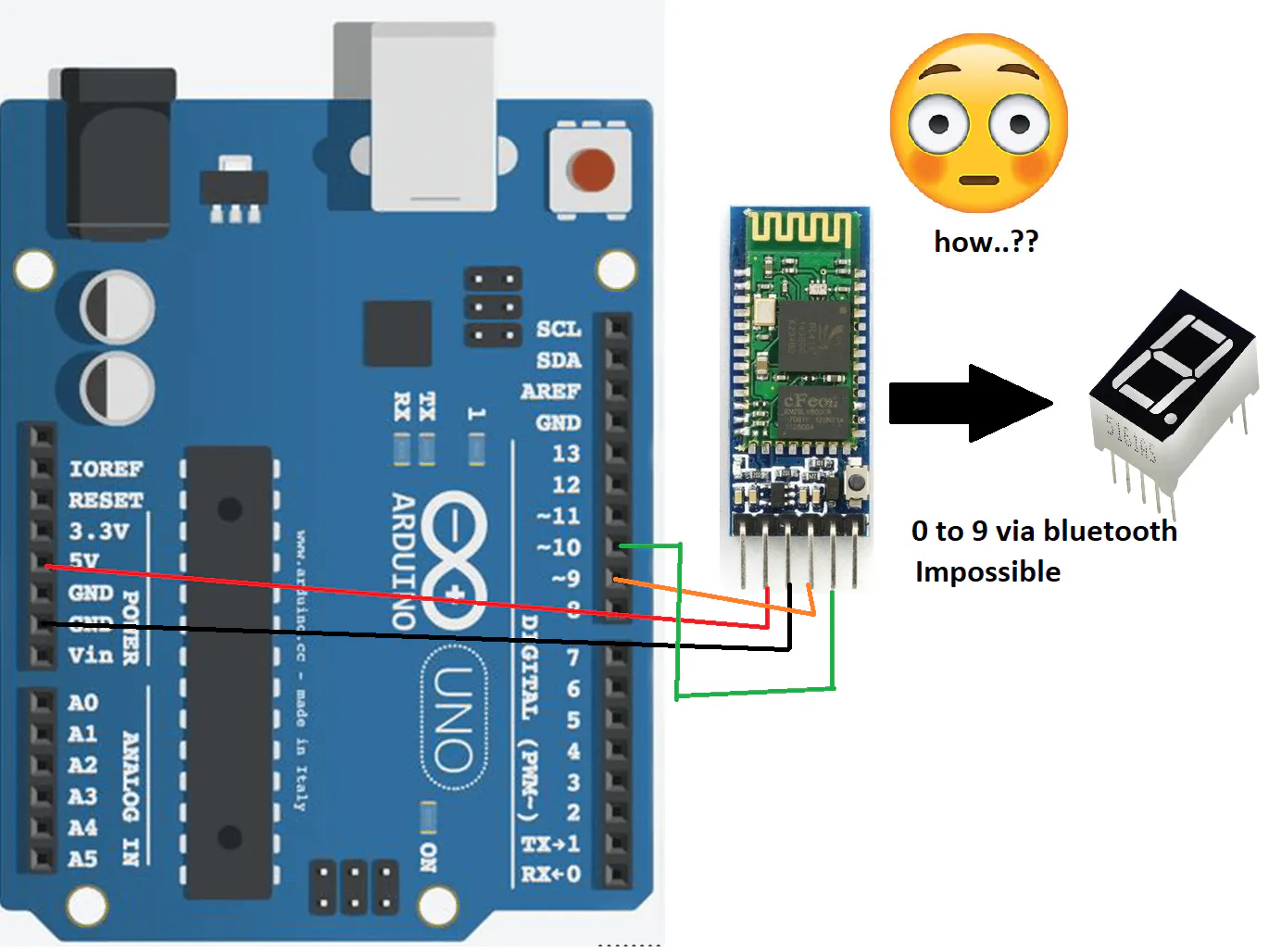## Things used in this project

### Hardware componentsArduino UNO & Genuino UNO
×1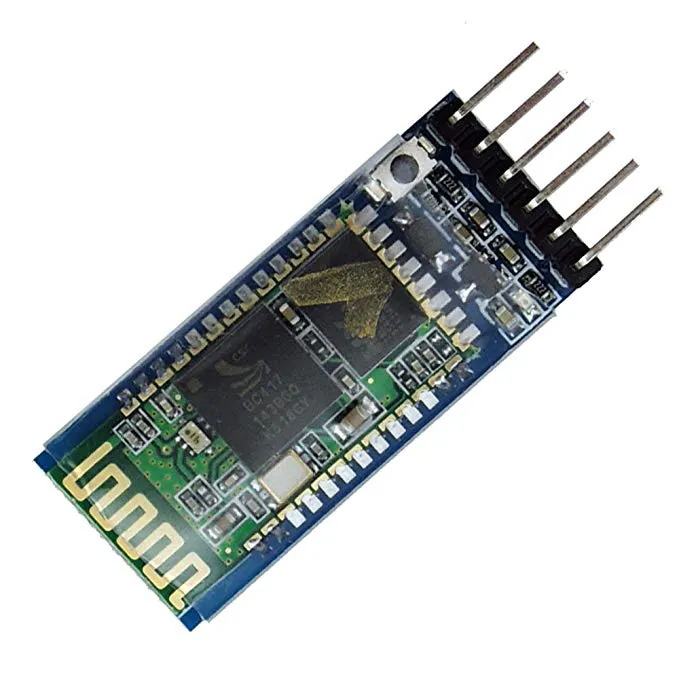HC-05 Bluetooth Module
×1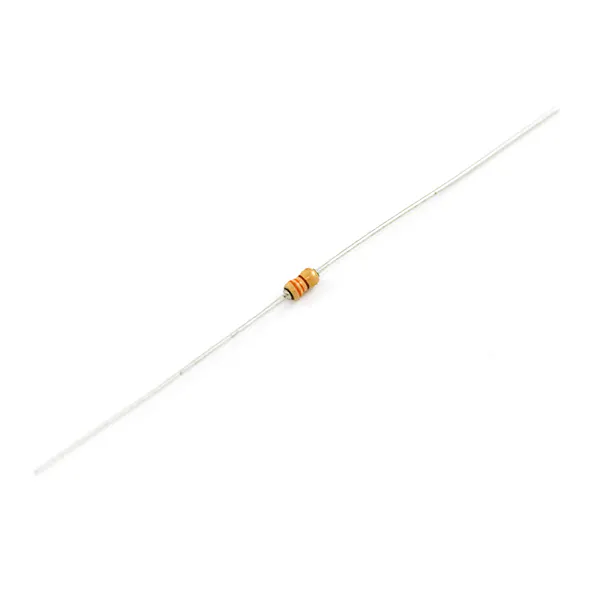Resistor 330 ohm
×1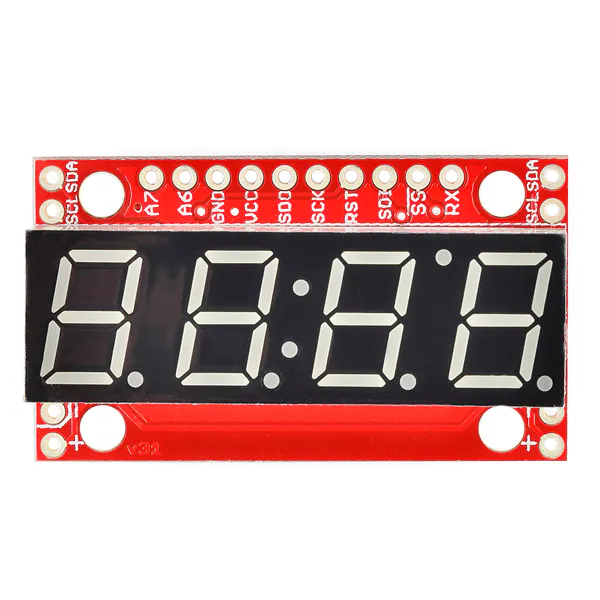SparkFun 7-Segment Serial Display - Red
×1×1Jumper wires (generic)
×1

### Software apps and online servicesArduino IDE

## Schematics

### Circuit Diagram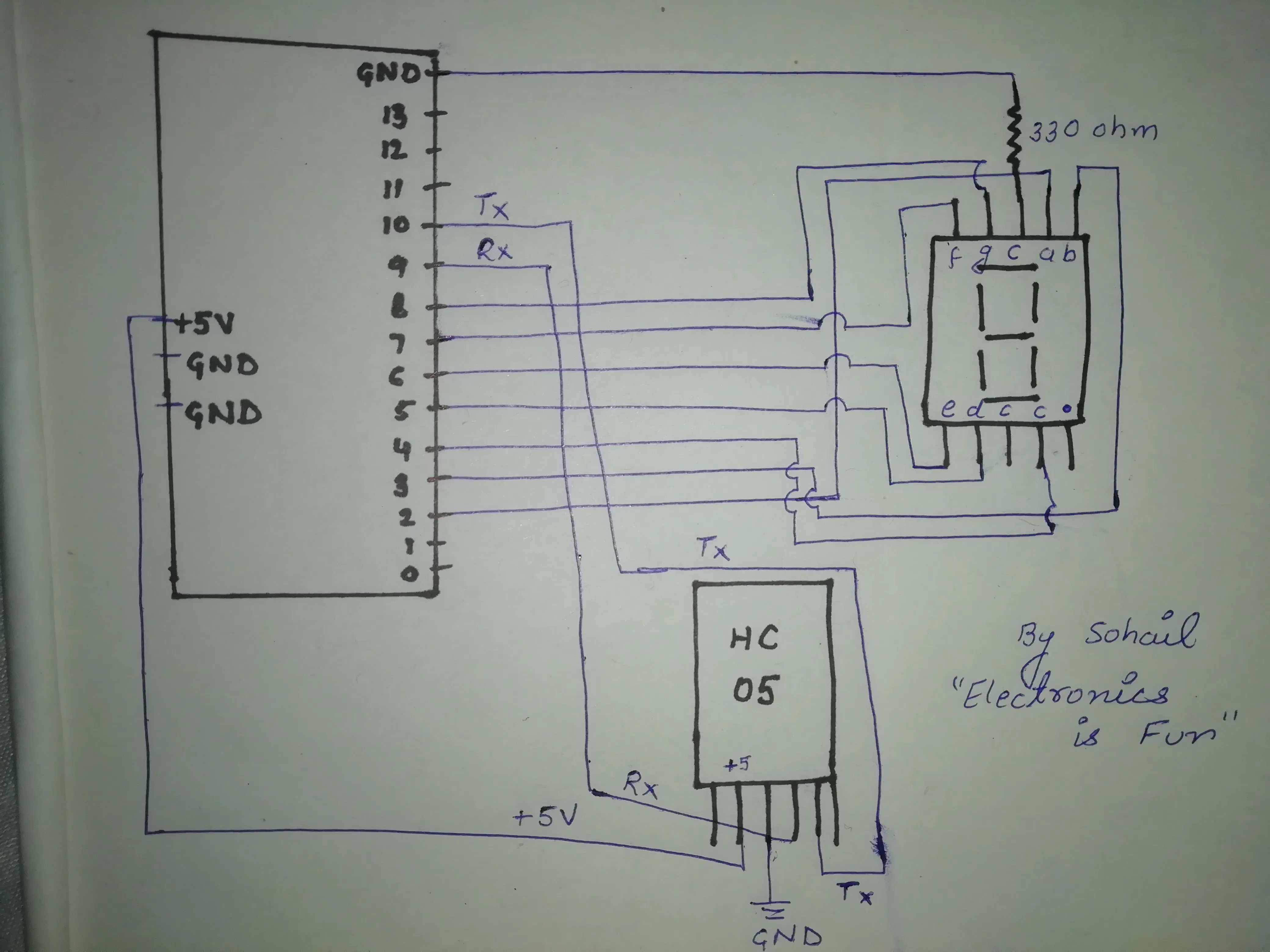## Code

### c

C/C++
```//www.youtube.com/Electronics is Fun//

#include<SoftwareSerial.h>

#define TxD 10
#define RxD 9
int a=2;
int b=3;
int h=4;
int d=5;
int e=6;
int f=7;
int g=8;

SoftwareSerial bluetoothSerial(TxD, RxD);

char c;

void setup() {
bluetoothSerial.begin(9600);
Serial.begin(9600);
pinMode(a, OUTPUT);
pinMode(b, OUTPUT);
pinMode(h, OUTPUT);
pinMode(d, OUTPUT);
pinMode(e, OUTPUT);
pinMode(f, OUTPUT);
pinMode(g, OUTPUT);
}

void loop() {
if(bluetoothSerial.available()){
Serial.println(c);
if(c=='0'){
digitalWrite(a,1);
digitalWrite(b,1);
digitalWrite(h,1);
digitalWrite(d,1);
digitalWrite(e,1);
digitalWrite(f,1);
digitalWrite(g,0);;
}
if(c=='1'){
digitalWrite(a,0);
digitalWrite(b,1);
digitalWrite(h,1);
digitalWrite(d,0);
digitalWrite(e,0);
digitalWrite(f,0);
digitalWrite(g,0);;
}
if(c=='2'){
digitalWrite(a,1);
digitalWrite(b,1);
digitalWrite(h,0);
digitalWrite(d,1);
digitalWrite(e,1);
digitalWrite(f,0);
digitalWrite(g,1);;
}
if(c=='3'){
digitalWrite(a,1);
digitalWrite(b,1);
digitalWrite(h,1);
digitalWrite(d,1);
digitalWrite(e,0);
digitalWrite(f,0);
digitalWrite(g,1);
}
if(c=='4'){
digitalWrite(a,0);
digitalWrite(b,1);
digitalWrite(h,1);
digitalWrite(d,0);
digitalWrite(e,0);
digitalWrite(f,1);
digitalWrite(g,1);
}
if(c=='5'){
digitalWrite(a,1);
digitalWrite(b,0);
digitalWrite(h,1);
digitalWrite(d,1);
digitalWrite(e,0);
digitalWrite(f,1);
digitalWrite(g,1);
}
if(c=='6'){
digitalWrite(a,1);
digitalWrite(b,0);
digitalWrite(h,1);
digitalWrite(d,1);
digitalWrite(e,1);
digitalWrite(f,1);
digitalWrite(g,1);
}
if(c=='7'){
digitalWrite(a,1);
digitalWrite(b,1);
digitalWrite(h,1);
digitalWrite(d,0);
digitalWrite(e,0);
digitalWrite(f,0);
digitalWrite(g,0);
}
if(c=='8'){
digitalWrite(a,1);
digitalWrite(b,1);
digitalWrite(h,1);
digitalWrite(d,1);
digitalWrite(e,1);
digitalWrite(f,1);
digitalWrite(g,1);
}
if(c=='9'){
digitalWrite(a,1);
digitalWrite(b,1);
digitalWrite(h,1);
digitalWrite(d,1);
digitalWrite(e,0);
digitalWrite(f,1);
digitalWrite(g,1);
}
}
}
```

## Credits

### msr048

0 projects • 49 followers